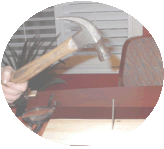#### Dynamics - The causes of Motion

Sir Isaac Newton defined and described the motion of objects using three very simple but powerful Laws.

##### The First Law is known as the Law of Inertia.

Inertia is a measure of the "idleness" of an object. It's the resistance of an object to move or to the change its state motion.

Newton's Law of Inertia states that an "Objects at rest will stay at rest or will continue to move in the same direction and with the same speed, unless an external (unbalanced) force acts upon them".

This simple animation demonstrates the Law of Inertia. A cart moving at constant speed is carrying a load.  The load is resting on top of the cart. When the cart hits the obstacle the load that is resting on it will continue to move at the same speed as the cart.Note that the cart bounces back - this will be explained later using Newton's Third Law - also known as  the "action-reaction" law.

Example:

If you are a passenger in a car cruising at a steady, comfortable speed of say 60 km/h and suddenly the driver hits the brakes -- what will happen?

You will continue to move forward at 60 km/h because you have the tendency to keep doing what you were doing before the brakes were applied, i.e. move at a constant speed of 60 Km/h.Passengers in a car are required to wear seatbelts.  In case the brakes are suddenly applied, the passengers will be restrained from moving forward when the action of the brakes is applying a backward motion. Not wearing seatbelts when driving or riding a car is not only unsafe but also illegal.

Newton's Second Law of Motion

This law of motion looks at the force that will force objects to change their state of rest or motion.  In soccer, the harder you kick the ball, the faster in moves (accelerates).  The ball moves in the direction of the kick.

If you kick a tennis ball with the same force as you would kick a soccer ball -- what happens?

The tennis ball will accelerate much faster -- why?  Because the tennis ball has less mass than the soccer ball.

Newton's Second Law states that the stronger the force acting on a mass, the greater its acceleration.  If the mass increases, the acceleration will decrease provided that the force stays constant.

This animation demonstrates the relationship between Force and AccelerationNote the effect that a 1 unit force has on the acceleration two different masses; where one mass is twice the value of the other mass.  The smaller mass moves twice the distance in the same time period.

The acceleration of an object is proportional to the unbalanced force and is inversely proportional to the mass of the object.  We call this "unbalanced" force, the net force Fnet .

In mathematical terms:

Net Force = mass X acceleration

Using Symbols:

Fnet = m x a

 Quantity Symbol Unit Net Force Fnet Newton [N] Mass m Kilogram [Kg] Acceleration a m/s2
##### The Newton -- a unit of force1 Newton [N] is approximately the force of gravity ("the weight") acting on a medium sized apple falling under the influence of gravity alone.1 Newton is also defined as the force that accelerates a 1 Kg mass at 1 m/s.Make sure that the units are always in Newtons, Kilograms, and meters-per-second-squared when you you use this formula1Newton can also be defined in terms of its fundamental units Kg/m/s21 N = 1 Kg/m/s2

Example:
What is the net force required to make a 300 g soccer ball accelerate at 2.0 m/s ?

Given:

• m = 300 g = 0.30 Kg
• a   = 2.0 m/s

Find:  Fnet

Solution:

Fnet = m X a

Fnet = 0.30 Kg X 2.0 m/s
= 0.60 N

##### The Third Law

This law of motion looks is also known as the action-reaction Law. "For every action, there exists an equal but opposite reaction".

Examples:

1. When rowing a boat one notices that the boat moves in the opposite direction of the motion of the oars.   In skating, you push back on the skates to move forward.
2. The recoil action of a rifle: as the bullet leaves the muzzle of the gun, the gun kicks back in the opposite direction.

The slow-motion film strip shows a nail being hammered by a hammer.This animation illustrates Newtons Third Law of Motion.  As the hammer strikes the nail with a force Faction [down], the nail strikes back with an opposite Freaction [up].